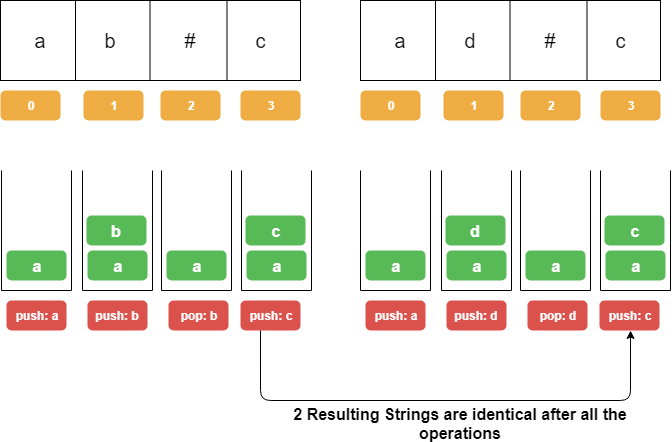## String Compare with Backspace operation

Given two strings `S` and `T`, return if they are equal when both are typed into empty text editors. `#` means a backspace character.

Example 1:

```Input: S = "ab#c", T = "ad#c"
Output: true
Explanation: Both S and T become "ac".
```

Example 2:

```Input: S = "ab##", T = "c#d#"
Output: true
Explanation: Both S and T become "".
```

Example 3:

```Input: S = "a##c", T = "#a#c"
Output: true
Explanation: Both S and T become "c".
```

Example 4:

```Input: S = "a#c", T = "b"
Output: false
Explanation: S becomes "c" while T becomes "b".
```

Note:

1. `1 <= S.length <= 200`
2. `1 <= T.length <= 200`
3. `S` and `T` only contain lowercase letters and `'#'` characters.

The problem can be solved using 2 `Stacks`. For each of the input String

1. We will push all characters one by one into the stack except '#' .
2. We will pop an element from the stack whenever we encountered a #, simulating the backspace operation.
3. In the end, we will check the content of both the `Stacks.`

Check The pictorial representation of the approach for input String S = "ab#c", T = "ad#c"Java Implementation:

``````class BackspaceCompareSolution {

public boolean backspaceCompare(String S, String T) {
Stack < Character > stack1 = getStack(S);
Stack < Character > stack2 = getStack(T);
return stack1.equals(stack2);
}

private Stack < Character > getStack(String s) {
Stack < Character > stack = new Stack < > ();
char arr[] = s.toCharArray();
for (char current: arr) {
if (current != '#') {
stack.push(current);
} else if (current == '#') {
if (!stack.isEmpty()) {
stack.pop();
}
}
}
return stack;
}
}``````

Algorithm String